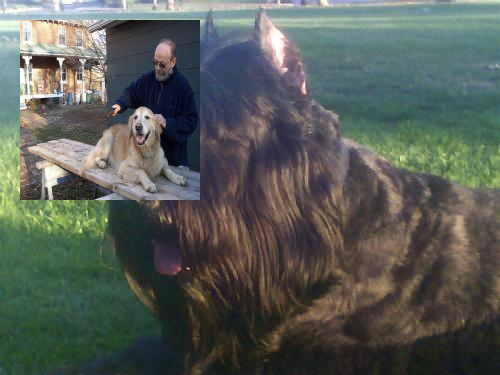# Spatial Examples¶

:

from easycv import Image
from easycv.transforms import Resize, Rescale, Rotate, Crop, Translate, Mirror, Paste


For this example we will load an image with random(). For more examples of image loading check Image examples.

:

img = Image.random()
img

:## Resize¶

To resize an image we simply apply the Rezise Transform, for more information check the reference.

:

img.apply(Resize(width=300, height=250))

: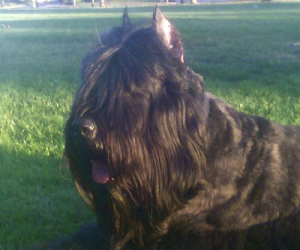## Rescale¶

To rescale an image we simply apply the Rescale Transform with the factors of x and y, for more information check the reference.

:

img.apply(Rescale(fx=2, fy=1))

:## Rotate¶

To rotate an image we simply apply the Rotate Transform with the degrees to rotate, for more information check the reference.

:

img.apply(Rotate(degrees=50))

: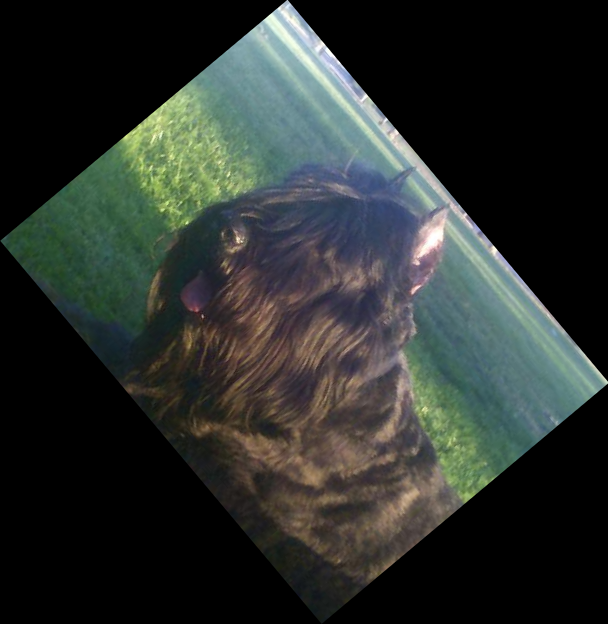## Crop¶

To crop an image we simply apply the Crop Transform with the boundaries of each side, for more information check the reference.

:

img.apply(Crop(rectangle=[[50,50],[100,100]]))

: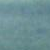## Translate¶

To translate an image we simply apply the Translate Transform with the vector to translate, for more information check the reference.

:

img.apply(Translate(x=10,y=50))

: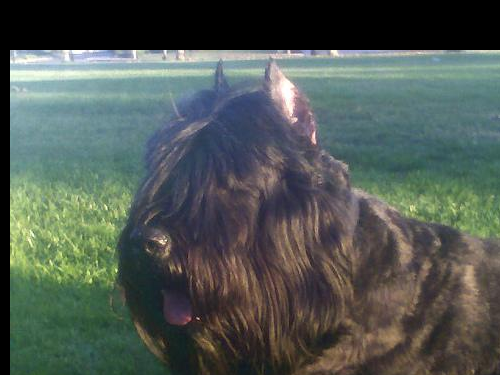## Mirror¶

To flip an image we simply apply the Mirror Transform with the desired axis to flip, for more information check the reference.

:

img.apply(Mirror(axis="y"))

: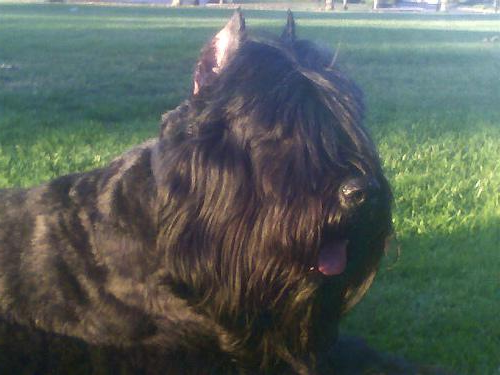## Paste¶

To paste an image on to another simply apply the Paste transform with the image to use and the rectangle to paste, for more information check the reference.

:

img.apply(Paste(paste=Image.random(), rectangle=[[20,20],[200,200]]))

: